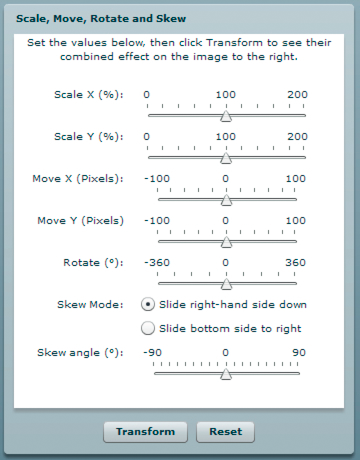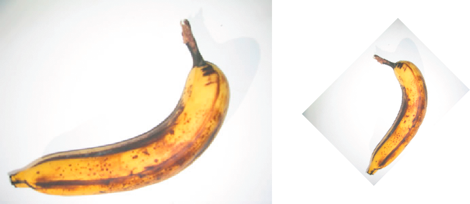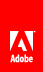# Geometry example: Applying a matrix transformation to a display object

Flash Player 9 and later, Adobe AIR 1.0 and later

The DisplayObjectTransformer sample application shows a number of features of using the Matrix class to transform a display object, including the following:

• Rotating the display object

• Scaling the display object

• Translating (repositioning) the display object

• Skewing the display object

The application provides an interface for adjusting the parameters of the matrix transformation, as follows:When the user clicks the Transform button, the application applies the appropriate transformation.The original display object, and the display object rotated by -45° and scaled by 50%

To get the application files for this sample, see www.adobe.com/go/learn_programmingAS3samples_flash . The DisplayObjectTransformer application files can be found in the folder Samples/DisplayObjectTransformer. The application consists of the following files:

File

Description

DisplayObjectTransformer.mxml

or

DisplayObjectTransformer.fla

The main application file in Flash (FLA) or Flex (MXML)

com/example/programmingas3/geometry/MatrixTransformer.as

A class that contains methods for applying matrix transformations.

img/

A directory containing sample image files used by the application.

## Defining the MatrixTransformer class

The MatrixTransformer class includes static methods that apply geometric transformations of Matrix objects.

#### The transform() method

The transform() method includes parameters for each of the following:

• sourceMatrix —The input matrix, which the method transforms

• xScale and yScale —The x and y scale factor

• dx and dy —The x and y translation amounts, in pixels

• rotation —The rotation amount, in degrees

• skew —The skew factor, as a percentage

• skewType —The direction in which the skew, either "right" or "left"

The return value is the resulting matrix.

The transform() method calls the following static methods of the class:

• skew()

• scale()

• translate()

• rotate()

Each returns the source matrix with the applied transformation.

#### The skew() method

The skew() method skews the matrix by adjusting the b and c properties of the matrix. An optional parameter, unit , determines the units used to define the skew angle, and if necessary, the method converts the angle value to radians:

```if (unit == "degrees")
{
angle = Math.PI * 2 * angle / 360;
}
{
angle = Math.PI * 2 * angle / 100;
}```

A skewMatrix Matrix object is created and adjusted to apply the skew transformation. Initially, it is the identity matrix, as follows:

`var skewMatrix:Matrix = new Matrix();`

The skewSide parameter determines the side to which the skew is applied. If it is set to "right" , the following code sets the b property of the matrix:

`skewMatrix.b = Math.tan(angle);`

Otherwise, the bottom side is skewed by adjusting the c property of the Matrix, as follows:

`skewMatrix.c = Math.tan(angle);`

The resulting skew is then applied to the existing matrix by concatenating the two matrixes, as the following example shows:

```sourceMatrix.concat(skewMatrix);
return sourceMatrix;```

#### The scale() method

The following example shows the scale() method adjusts the scale factor if it is provided as a percentage, first, and then uses the scale() method of the matrix object:

```if (percent)
{
xScale = xScale / 100;
yScale = yScale / 100;
}
sourceMatrix.scale(xScale, yScale);
return sourceMatrix;```

#### The translate() method

The translate() method simply applies the dx and dy translation factors by calling the translate() method of the matrix object, as follows:

```sourceMatrix.translate(dx, dy);
return sourceMatrix;```

#### The rotate() method

The rotate() method converts the input rotation factor to radians (if it is provided in degrees or gradients), and then calls the rotate() method of the matrix object:

```if (unit == "degrees")
{
angle = Math.PI * 2 * angle / 360;
}
{
angle = Math.PI * 2 * angle / 100;
}
sourceMatrix.rotate(angle);
return sourceMatrix;```

## Calling the MatrixTransformer.transform() method from the application

The application contains a user interface for getting the transformation parameters from the user. It then passes these values, along with the matrix property of the transform property of the display object, to the Matrix.transform() method, as follows:

```tempMatrix = MatrixTransformer.transform(tempMatrix,
xScaleSlider.value,
yScaleSlider.value,
dxSlider.value,
dySlider.value,
rotationSlider.value,
skewSlider.value,
skewSide );```

The application then applies the return value to the matrix property of the transform property of the display object, triggering the transformation:

`img.content.transform.matrix = tempMatrix;`// Ethnio survey code removed